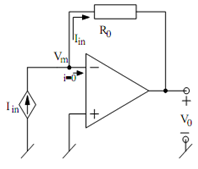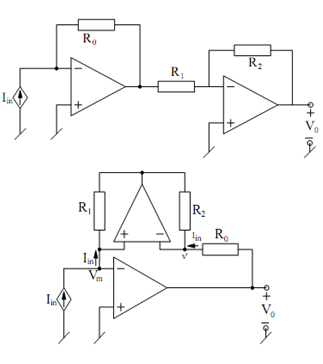## Current Controlled Voltage Sources Assignment Help

Assignment Help: >> Basic Analog Circuits using Ideal Op-amps - Current Controlled Voltage Sources

Current Controlled Voltage Sources:

The circuit is illustrated in Figure, since, non inverting input terminal is connected to ground, because of infinite gain and negative feedback, the inverting input terminal is forced to have ground potential (virtual ground) because of which Vm = 0.Figure: Inverting Current Controlled Voltage Source

Also, because infinite Zin, no current is drawn by the op-amp input terminal.

Hence,

Iin  = 0 - V0 / R0

or         V0  = - R0 Iin

and the circuit acts as an inverting CCVS.

Non-inverting CCVS can be obtained in two different ways as illustrated in FigureFigure: Non-inverting CCVS Structures

(i)   By cascading an inverting VCVS with the circuit of a inverting CCVS, so that

V0  =- (R0  Iin)(-R2 /R1)   = + (R0 R 2)Iin or

(ii) By putting a NIC with in the feedback path of the inverting CCVS, the analysis for which gives

V0        = +( R1 R0 / R2)Iin